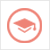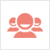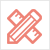﻿ Essay范文-econ essay-51Due留学教育

#### 服务承诺资金托管原创保证实力保障24小时客服使命必达

#### 关于我们

51Due提供Essay，Paper，Report，Assignment等学科作业的代写与辅导，同时涵盖Personal Statement，转学申请等留学文书代写。51Due将让你达成学业目标51Due将让你达成学业目标51Due将让你达成学业目标51Due将让你达成学业目标

#### 名企实习积累工作经验多元化文化交流专业实操技能建立人际资源圈

# econ essay

2021-04-24 来源: 51Due教员组 类别: Essay范文

51Due教员组今天给各位留学生带来一篇经济代写范文（econ essay）。

(i) With the aid of appropriate diagrams, explain why indifference curves are traditionally convex to the origin. (10%)

If we assume that the curve is sloping up, it violates the assumption that any commodity is more than less. In the coordinates, the market basket combination on the upper right must be more preferred than the basket on the lower left, because at this time, the amount of X and Y is increased, more products are obtained, and the utility of the consumer will increase. Assuming the indifference curve is convex toward the origin, it shows that the preferences of consumers who buy more goods are the same as the preferences of fewer goods, which violates the assumption that more is better than less.

The indifference curve is convex because of the hypothesis that the marginal substitution rate decreases. This means that with the increasing consumption of one commodity X, the number of consumers willing to give up the second commodity Y in order to obtain an additional unit of commodity X will decrease, which is a reasonable assumption. The slop of the indifference curve has other name called marginal substitution rate. Convex means that the slope is reduced. That is, the amount of change in the vertical axis caused by the change in one unit of the horizontal axis is decreasing, that is, the number of products in the vertical axis that are willing to give up to obtain one unit of the horizontal axis is reduced, so the figure is convex.

To sum up, the shape of the indifference curve depends on the two premise assumptions that the goods in the consumer behavior theory are better than less and the marginal substitution rate is decreasing. In reality, different situations may occur, but in order to study most of the economy Phenomena, we must set hypothetical conditions in order to study more accurately. So the conventional assumption of indifference curves is that indifference curves are traditionally convex to the origin.

(ii) Given the indifference curve for two-goods is convex, derive a price inelastic demand curve for Good X (10%).

Given the assumption that people's income and commodity prices are determined, people's income and commodity prices are unchanged, people's total income can buy different quantity combinations of goods and Y. By plotting the points of these product combinations, we can get the budget constraint line. For example, if I represents the consumer's money income, if all is used to purchase Y, then I = PY · Y; if all is used to purchase X, I = PX · X. Obviously, I = PX · X + PY · Y. The geometric figure is expressed as a straight line inclined from the top left to bottom right. The horizontal axis represents the purchase quantity of the product X, and the vertical axis represents the quantity of the product Y, which the consumer can buy. The absolute value of the slope of the budget line equals to PX / PY, which can be calculated by using the price of product X to divided by the price of product Y.

Given the assumption that we know the income of consumer and product prices, their amount is constant, which means there is no change. Then we can easily to draw the budget line. At the same time, if the consumer's preference is constant, it means that the consumer's indifference curve is also constant.  We place the consumer's budget line and the indifference curve in one figure together, and there a tangent point of the indifference curve and the budget constraint line we can found, and this point is the point called the maximum utility point obtained by consumer, we also call this is the equilibrium point of the consumer.

The horizontal axis is the number of X products, and the vertical axis is the number of Y products. When the price of X products decreases, such as from P1 to P3, the budget line uses the intersection on the vertical axis as the axis to go outward on the horizontal axis Expansion, tangent to higher-level indifference curves to achieve a new consumer equilibrium. It can be seen that the demand quantity of X product is increasing, from Z1 to Z3. We represent the price of X product and the corresponding demand quantity of  product X at each consumer equilibrium point in a coordinate system, with the price of X commodity as the vertical axis and the demand quantity of product X as the horizontal axis, and we get the demand curve of product X. We can find that the demand curve for product X is also a straight line inclined from top left to bottom right, which means as the price of X Good decreases, the demand quantity of product X increase.

iii）With reference to the previous elements of this coursework question, discuss the links between the indifference curve, price elasticity estimates and the theory of supply and demand in economics (80%).

Through the above question 1 and 2 ,we can derive the demand curve that slope from top of the left to the bottom of the right, based on the indifference curve and the budget constraint line. The demand curve is a curve showing the relationship between price and demand, and refers to the curve of the quantity of goods that people are willing to buy at different price levels under the same conditions. The flatness or steepness of the demand curve is determined by the slope. And steep and flat can be shown as the price elasticity of commodity demand.

Price elasticity of demand  (Ep) refers to the degree of response or sensitivity of demand (Q) to changes in price (P). That is Ep=（ΔP／P）/(ΔQ／Q).

(1) Ep＝1(Price elasticity demand). It shows that the change in demand is the same as the price.;

(2) 1 <Ep <∝ (elastic). It shows that the change in demand is greater than the price, and the demand curve is relatively flat (the slope is small);

(3) 0 <Ep <1 (inelastic). It means that the change in demand is smaller than the price change. The demand curve is steeper (the slope is larger);

(4) Ep → 0 (perfect inelastic). At this time, the demand does not change with the price. The demand curve is a straight line perpendicular to the abscissa;

5) Ep → ∝ (perfect elastic). At this time, the demand can be arbitrarily changed under the given price. The demand curve will be a straight line parallel to the abscissa;

The supply curve is a curve showing the relationship between the price and the supply quantity. It refers to the curve where the supplier is willing to supply the quantity of goods at different price levels under the same conditions. Generally, the higher the price, the more supply, so the curve goes to the upper right Square tilt. The main factors affecting supply are: the price of the commodity itself and related commodities, the cost of production, the level of technology and so on.

Place the demand curve and supply curve in the same coordinate system to When the demand curve and the supply curve are analyzed together, the two curves will generate an intersection point, which will correspond to a price. At this time, the demand price is equal to the supply price, the price is the market equilibrium price, and the output corresponding to the intersection point. The demand output is the supply output, the output is the market equilibrium output, and this point is the market equilibrium point. Therefore, the demand curve and the supply curve jointly determine the market equilibrium point. If the price of a product is higher than the price of equilibrium point, the supply of the product will exceed the demand, and products will start to fall behind; in order to clear the inventory, the seller must begin to reduce prices until people are willing to buy. As a result, prices began to fall towards this equilibrium point, making supply equals to demand. If the price of commodities falls below the equilibrium point, then the demand will exceed the supply. At this time, people often line up to buy the product. When suppliers find this phenomenon, they will start to raise prices, and as a result, demand will fall, and supply will start to rise, until the two quantities are equal again, and the price reaches an equilibrium point. The position of the equilibrium point has its specific economic significance: price and quantity are efficient without causing waste.

In summary, if supply exceeds demand, the price of the product will fall; if supply provided is more than the quantity of demand people need, the price of the product will rise.

However, the price of the product we analyzed is essentially determined by value, so the impact of supply and demand on prices cannot seriously deviate from the value of the commodity. Moreover, the effect of supply and demand on prices is short term impact. Because oversupply will cause producers to consider it unprofitable and reduce the scale of production. In this way, it will lead to reduce supply and the price will rise again. When demand is more that supply, the producer will be driven by the benefits to expand the scale of production, which will lead to oversupply. Prices will fall again. Therefore, the theory of supply and demand affects the output and price levels of the entire society.

51due留学教育原创版权郑重声明：原创优秀代写范文源自编辑创作，未经官方许可，网站谢绝转载。对于侵权行为，未经同意的情况下，51Due有权追究法律责任。主要业务有essay代写assignment代写paper代写作业代写、论文代写服务。

51due为留学生提供最好的论文代写服务，亲们可以进入主页了解和获取更多代写范文提供论文代写服务，详情可以咨询我们的客服QQ：800020041。